summary of site-wide JavaScript functionalityUnited States-English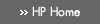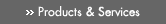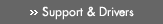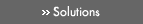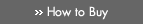» Contact HPSearch:More optionsManualTechnical documentation - EnglishAll of HP USHP-UX Reference > Part IV Section 3: Library Functions# C»

## Technical documentation» Feedback## » Indexcabs(3M) - complex absolute value (also called norm, modulus, or magnitude) functions
cabsf(3M) - complex absolute value (also called norm, modulus, or magnitude) functions — see cabs(3M)
cabsl(3M) - complex absolute value (also called norm, modulus, or magnitude) functions — see cabs(3M)
cabsq(3M) - complex absolute value (also called norm, modulus, or magnitude) functions — see cabs(3M)
cabsw(3M) - complex absolute value (also called norm, modulus, or magnitude) functions — see cabs(3M)
cacos(3M) - complex arccosine functions
cacosf(3M) - complex arccosine functions — see cacos(3M)
cacosh(3M) - complex arc hyperbolic cosine functions
cacoshf(3M) - complex arc hyperbolic cosine functions — see cacosh(3M)
cacoshl(3M) - complex arc hyperbolic cosine functions — see cacosh(3M)
cacoshq(3M) - complex arc hyperbolic cosine functions — see cacosh(3M)
cacoshw(3M) - complex arc hyperbolic cosine functions — see cacosh(3M)
cacosl(3M) - complex arccosine functions — see cacos(3M)
cacosq(3M) - complex arccosine functions — see cacos(3M)
cacosw(3M) - complex arccosine functions — see cacos(3M)
calloc(3C) - main memory allocator — see malloc(3C)
callrpc(3N) - obsolete library routines for RPC — see rpc_soc(3N)
can_change_color(3X) - color manipulation functions
carg(3M) - complex argument (also called phase angle) functions
cargf(3M) - complex argument (also called phase angle) functions — see carg(3M)
cargl(3M) - complex argument (also called phase angle) functions — see carg(3M)
cargq(3M) - complex argument (also called phase angle) functions — see carg(3M)
cargw(3M) - complex argument (also called phase angle) functions — see carg(3M)
casin(3M) - complex arcsine functions
casinf(3M) - complex arcsine functions — see casin(3M)
casinh(3M) - complex arc hyperbolic sine functions
casinhf(3M) - complex arc hyperbolic sine functions — see casinh(3M)
casinhl(3M) - complex arc hyperbolic sine functions — see casinh(3M)
casinhq(3M) - complex arc hyperbolic sine functions — see casinh(3M)
casinhw(3M) - complex arc hyperbolic sine functions — see casinh(3M)
casinl(3M) - complex arcsine functions — see casin(3M)
casinq(3M) - complex arcsine functions — see casin(3M)
casinw(3M) - complex arcsine functions — see casin(3M)
catan(3M) - complex arctangent functions
catanf(3M) - complex arctangent functions — see catan(3M)
catanh(3M) - complex arc hyperbolic tangent functions
catanhf(3M) - complex arc hyperbolic tangent functions — see catanh(3M)
catanhl(3M) - complex arc hyperbolic tangent functions — see catanh(3M)
catanhq(3M) - complex arc hyperbolic tangent functions — see catanh(3M)
catanhw(3M) - complex arc hyperbolic tangent functions — see catanh(3M)
catanl(3M) - complex arctangent functions — see catan(3M)
catanq(3M) - complex arctangent functions — see catan(3M)
catanw(3M) - complex arctangent functions — see catan(3M)
catclose(3C) - open and close a message catalog for reading — see catopen(3C)
catgets(3C) - get a program message
catopen(3C) - open and close a message catalog for reading
cbreak(3X) - input mode control functions
cbrt(3M) - cube root functions
cbrtf(3M) - cube root functions — see cbrt(3M)
cbrtl(3M) - cube root functions — see cbrt(3M)
cbrtq(3M) - cube root functions — see cbrt(3M)
cbrtw(3M) - cube root functions — see cbrt(3M)
ccos(3M) - complex cosine functions
ccosf(3M) - complex cosine functions — see ccos(3M)
ccosh(3M) - complex hyperbolic cosine functions
ccoshf(3M) - complex hyperbolic cosine functions — see ccosh(3M)
ccoshl(3M) - complex hyperbolic cosine functions — see ccosh(3M)
ccoshq(3M) - complex hyperbolic cosine functions — see ccosh(3M)
ccoshw(3M) - complex hyperbolic cosine functions — see ccosh(3M)
ccosl(3M) - complex cosine functions — see ccos(3M)
ccosq(3M) - complex cosine functions — see ccos(3M)
ccosw(3M) - complex cosine functions — see ccos(3M)
ceil(3M) - ceiling functions
ceilf(3M) - ceiling functions — see ceil(3M)
ceill(3M) - ceiling functions — see ceil(3M)
ceilq(3M) - ceiling functions — see ceil(3M)
ceilw(3M) - ceiling functions — see ceil(3M)
cexp(3M) - complex exponential functions
cexpf(3M) - complex exponential functions — see cexp(3M)
cexpl(3M) - complex exponential functions — see cexp(3M)
cexpq(3M) - complex exponential functions — see cexp(3M)
cexpw(3M) - complex exponential functions — see cexp(3M)
cfgetispeed(3C) - tty baud rate functions — see cfspeed(3C)
cfgetospeed(3C) - tty baud rate functions — see cfspeed(3C)
cfsetispeed(3C) - tty baud rate functions — see cfspeed(3C)
cfsetospeed(3C) - tty baud rate functions — see cfspeed(3C)
cfspeed(3C) - tty baud rate functions
chgat(3X) - change renditions of characters in a window
chownacl(3C) - change owner and/or group represented in a file's access control list (ACL) (HFS File Systems only)
cimag(3M) - the imaginary part of a complex value
cimagf(3M) - the imaginary part of a complex value — see cimag(3M)
cimagl(3M) - the imaginary part of a complex value — see cimag(3M)
cimagq(3M) - the imaginary part of a complex value — see cimag(3M)
cimagw(3M) - the imaginary part of a complex value — see cimag(3M)
cis(3M) - complex value with unit magnitude and given angle (specified in radians)
cisf(3M) - complex value with unit magnitude and given angle (specified in radians) — see cis(3M)
cisl(3M) - complex value with unit magnitude and given angle (specified in radians) — see cis(3M)
cisq(3M) - complex value with unit magnitude and given angle (specified in radians) — see cis(3M)
cisw(3M) - complex value with unit magnitude and given angle (specified in radians) — see cis(3M)
clear(3X) - clear a window
clearenv(3C) - clear the process environment
clearerr(3S) - stream status inquiries — see ferror(3S)
clearerr_unlocked(3S) - stream status inquiries — see ferror(3S)
clearok(3X) - terminal output control functions
clnt_broadcast(3N) - obsolete library routines for RPC — see rpc_soc(3N)
clnt_call(3N) - library routines for client side calls — see rpc_clnt_calls(3N)
clnt_control(3N) - library routines for dealing with creation and manipulation of CLIENT handles — see rpc_clnt_create(3N)
clnt_create(3N) - library routines for dealing with creation and manipulation of CLIENT handles — see rpc_clnt_create(3N)
clnt_create_vers(3N) - library routines for dealing with creation and manipulation of CLIENT handles — see rpc_clnt_create(3N)
clnt_destroy(3N) - library routines for dealing with creation and manipulation of CLIENT handles — see rpc_clnt_create(3N)
clnt_dg_create(3N) - library routines for dealing with creation and manipulation of CLIENT handles — see rpc_clnt_create(3N)
clnt_freeres(3N) - library routines for client side calls — see rpc_clnt_calls(3N)
clnt_geterr(3N) - library routines for client side calls — see rpc_clnt_calls(3N)
clnt_pcreateerror(3N) - library routines for dealing with creation and manipulation of CLIENT handles — see rpc_clnt_create(3N)
clnt_perrno(3N) - library routines for client side calls — see rpc_clnt_calls(3N)
clnt_perror(3N) - library routines for client side calls — see rpc_clnt_calls(3N)
clnt_raw_create(3N) - library routines for dealing with creation and manipulation of CLIENT handles — see rpc_clnt_create(3N)
clnt_spcreateerror(3N) - library routines for dealing with creation and manipulation of CLIENT handles — see rpc_clnt_create(3N)
clnt_sperrno(3N) - library routines for client side calls — see rpc_clnt_calls(3N)
clnt_sperror(3N) - library routines for client side calls — see rpc_clnt_calls(3N)
clnt_tli_create(3N) - library routines for dealing with creation and manipulation of CLIENT handles — see rpc_clnt_create(3N)
clnt_tp_create(3N) - library routines for dealing with creation and manipulation of CLIENT handles — see rpc_clnt_create(3N)
clnt_vc_create(3N) - library routines for dealing with creation and manipulation of CLIENT handles — see rpc_clnt_create(3N)
clntraw_create(3N) - obsolete library routines for RPC — see rpc_soc(3N)
clnttcp_create(3N) - obsolete library routines for RPC — see rpc_soc(3N)
clntudp_bufcreate(3N) - obsolete library routines for RPC — see rpc_soc(3N)
clntupd_create(3N) - obsolete library routines for RPC — see rpc_soc(3N)
clock(3C) - report CPU time used
clog(3M) - complex natural (base-e) logarithm functions
clogf(3M) - complex natural (base-e) logarithm functions — see clog(3M)
clogl(3M) - complex natural (base-e) logarithm functions — see clog(3M)
clogq(3M) - complex natural (base-e) logarithm functions — see clog(3M)
clogw(3M) - complex natural (base-e) logarithm functions — see clog(3M)
close_secdef(3) - security defaults configuration file routines — see secdef(3)
closedir(3C) - directory operations — see directory(3C)
closelog(3C) - control system log — see syslog(3C)
clrtobot(3X) - clear from cursor to end of window
clrtoeol(3X) - clear from cursor to end of line
cmpt_change(3) - set and get process' compartment
cmpt_endent(3) - map compartment name to number or number to name — see cmpt_getbynum(3)
cmpt_get(3) - set and get process' compartment — see cmpt_change(3)
cmpt_get_addrcid(3) - get the compartment IDs associated with a network interfaces — see cmpt_get_ifcid(3)
cmpt_get_endpoint_cid(3) - get the compartment IDs for INET domain communications. — see cmpt_get_peer_cid(3)
cmpt_get_ifcid(3) - get the compartment IDs associated with a network interfaces
cmpt_get_peer_cid(3) - get the compartment IDs for INET domain communications.
cmpt_getbyname(3) - map compartment name to number or number to name — see cmpt_getbynum(3)
cmpt_getbynum(3) - map compartment name to number or number to name
cmpt_getent(3) - map compartment name to number or number to name — see cmpt_getbynum(3)
cmpt_setent(3) - map compartment name to number or number to name — see cmpt_getbynum(3)
color_content(3X) - color manipulation functions — see can_change_color(3X)
color_set(3X) - window attribute control functions — see attr_get(3X)
COLS(3X) - number of columns on terminal screen
compile(3X) - regular expression compile and match routines — see regexp(3X)
compound(3M) - compound interest factor
compoundf(3M) - compound interest factor — see compound(3M)
compoundl(3M) - compound interest factor — see compound(3M)
compoundq(3M) - compound interest factor — see compound(3M)
compoundw(3M) - compound interest factor — see compound(3M)
confstr(3C) - get string-valued configuration values
conj(3M) - complex conjugate functions
conjf(3M) - complex conjugate functions — see conj(3M)
conjl(3M) - complex conjugate functions — see conj(3M)
conjq(3M) - complex conjugate functions — see conj(3M)
conjw(3M) - complex conjugate functions — see conj(3M)
conv(3C) - translate characters
copydvagent(3) - manipulate device assignment database entry for a trusted system — see getdvagent(3)
copylist(3G) - copy a file into memory
copysign(3M) - copysign functions
copysignf(3M) - copysign functions — see copysign(3M)
copysignl(3M) - copysign functions — see copysign(3M)
copysignq(3M) - copysign functions — see copysign(3M)
copysignw(3M) - copysign functions — see copysign(3M)
copywin(3X) - copy a region of a window
cos(3M) - cosine functions
cosd(3M) - cosine functions of an argument specified in degrees
cosdf(3M) - cosine functions of an argument specified in degrees — see cosd(3M)
cosdl(3M) - cosine functions of an argument specified in degrees — see cosd(3M)
cosdq(3M) - cosine functions of an argument specified in degrees — see cosd(3M)
cosdw(3M) - cosine functions of an argument specified in degrees — see cosd(3M)
cosf(3M) - cosine functions — see cos(3M)
cosh(3M) - hyperbolic cosine functions
coshf(3M) - hyperbolic cosine functions — see cosh(3M)
coshl(3M) - hyperbolic cosine functions — see cosh(3M)
coshq(3M) - hyperbolic cosine functions — see cosh(3M)
coshw(3M) - hyperbolic cosine functions — see cosh(3M)
cosl(3M) - cosine functions — see cos(3M)
cosq(3M) - cosine functions — see cos(3M)
cosw(3M) - cosine functions — see cos(3M)
cot(3M) - cotangent functions
cotd(3M) - cotangent functions of an argument specified in degrees
cotdf(3M) - cotangent functions of an argument specified in degrees — see cotd(3M)
cotdl(3M) - cotangent functions of an argument specified in degrees — see cotd(3M)
cotdq(3M) - cotangent functions of an argument specified in degrees — see cotd(3M)
cotdw(3M) - cotangent functions of an argument specified in degrees — see cotd(3M)
cotf(3M) - cotangent functions — see cot(3M)
cotl(3M) - cotangent functions — see cot(3M)
cotq(3M) - cotangent functions — see cot(3M)
cotw(3M) - cotangent functions — see cot(3M)
cpacl(3C) - copy the access control list (ACL) and mode bits from one file to another (HFS and JFS File Systems only)
cpow(3M) - complex power functions
cpowf(3M) - complex power functions — see cpow(3M)
cpowl(3M) - complex power functions — see cpow(3M)
cpowq(3M) - complex power functions — see cpow(3M)
cpoww(3M) - complex power functions — see cpow(3M)
cproj(3M) - functions that project all infinities onto the one on the positive real axis
cprojf(3M) - functions that project all infinities onto the one on the positive real axis — see cproj(3M)
cprojl(3M) - functions that project all infinities onto the one on the positive real axis — see cproj(3M)
cprojq(3M) - functions that project all infinities onto the one on the positive real axis — see cproj(3M)
cprojw(3M) - functions that project all infinities onto the one on the positive real axis — see cproj(3M)
cr_close(3) - close a crash dump descriptor
cr_info(3) - retrieve crash dump information
cr_isaddr(3) - validate whether physical page number was dumped
cr_open(3) - open crash dump for reading
cr_perror(3) - print a libcrash error or warning message
cr_set_node(3) - set node number
cr_uncompress(3) - uncompress a file in a crash dump
cr_verify(3) - verify integrity of crash dump
creal(3M) - the real part of a complex value
crealf(3M) - the real part of a complex value — see creal(3M)
creall(3M) - the real part of a complex value — see creal(3M)
crealq(3M) - the real part of a complex value — see creal(3M)
crealw(3M) - the real part of a complex value — see creal(3M)
crt0(3) - execution startup routines
crt0_ia(3) - execution startup routines for Integrity systems
crt0.o(3) - execution startup routines — see crt0(3)
crt0_pa(3) - execution startup routines for PA-RISC systems
crypt(3C) - generate hashing encryption
crypt_r(3C) - generate hashing encryption — see crypt(3C)
csin(3M) - complex sine functions
csinf(3M) - complex sine functions — see csin(3M)
csinh(3M) - complex hyperbolic sine functions
csinhf(3M) - complex hyperbolic sine functions — see csinh(3M)
csinhl(3M) - complex hyperbolic sine functions — see csinh(3M)
csinhq(3M) - complex hyperbolic sine functions — see csinh(3M)
csinhw(3M) - complex hyperbolic sine functions — see csinh(3M)
csinl(3M) - complex sine functions — see csin(3M)
csinq(3M) - complex sine functions — see csin(3M)
csinw(3M) - complex sine functions — see csin(3M)
csqrt(3M) - complex square root functions
csqrtf(3M) - complex square root functions — see csqrt(3M)
csqrtl(3M) - complex square root functions — see csqrt(3M)
csqrtq(3M) - complex square root functions — see csqrt(3M)
csqrtw(3M) - complex square root functions — see csqrt(3M)
ctan(3M) - complex tangent functions
ctanf(3M) - complex tangent functions — see ctan(3M)
ctanh(3M) - complex hyperbolic tangent functions
ctanhf(3M) - complex hyperbolic tangent functions — see ctanh(3M)
ctanhl(3M) - complex hyperbolic tangent functions — see ctanh(3M)
ctanhq(3M) - complex hyperbolic tangent functions — see ctanh(3M)
ctanhw(3M) - complex hyperbolic tangent functions — see ctanh(3M)
ctanl(3M) - complex tangent functions — see ctan(3M)
ctanq(3M) - complex tangent functions — see ctan(3M)
ctanw(3M) - complex tangent functions — see ctan(3M)
ctermid(3S) - generate file name for terminal
ctime(3C) - convert date and time to string
ctime_r(3C) - convert date and time to string — see ctime(3C)
ctype(3C) - classify characters according to type
cur_term(3X) - current terminal information
curs_set(3X) - set the cursor mode
curscr(3X) - current window
curses_intro(3X) - terminal and printer handling and optimization package
cuserid(3S) - get character login name of the user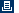Printable versionPrivacy statement Using this site means you accept its terms Feedback to webmaster© 1983-2007 Hewlett-Packard Development Company, L.P.